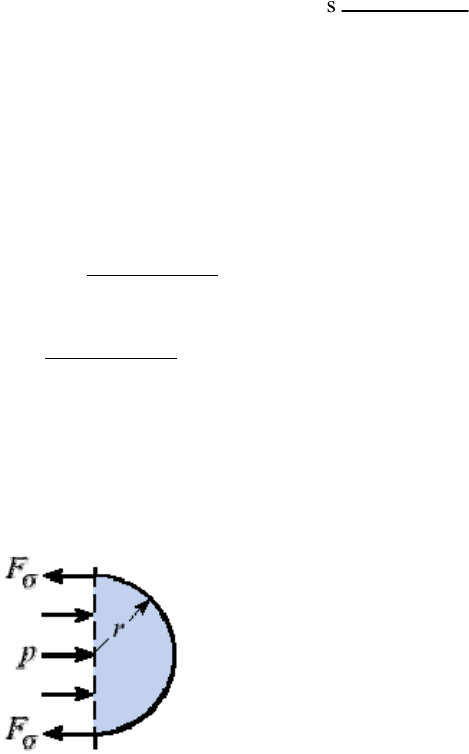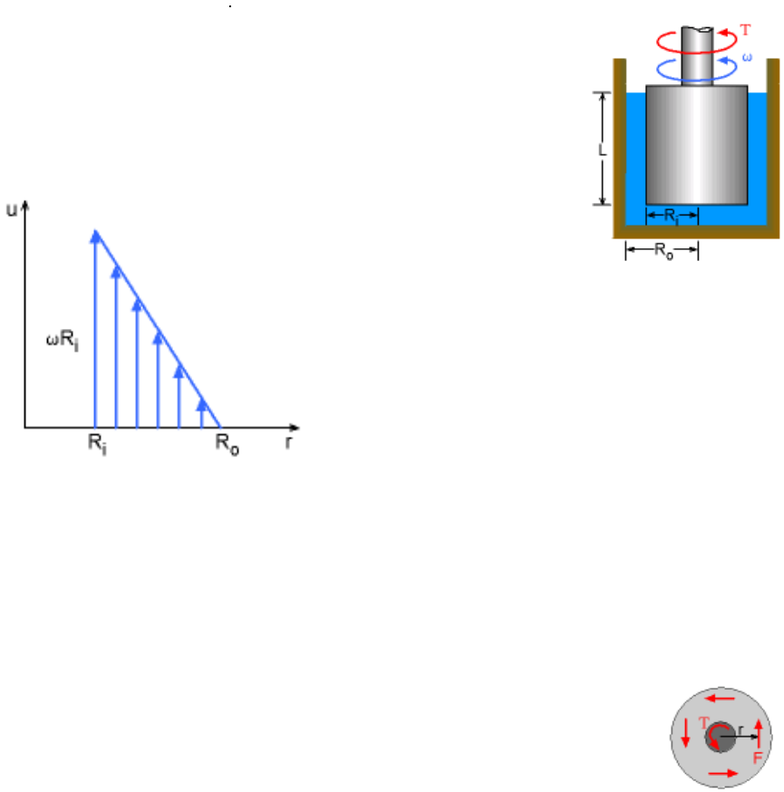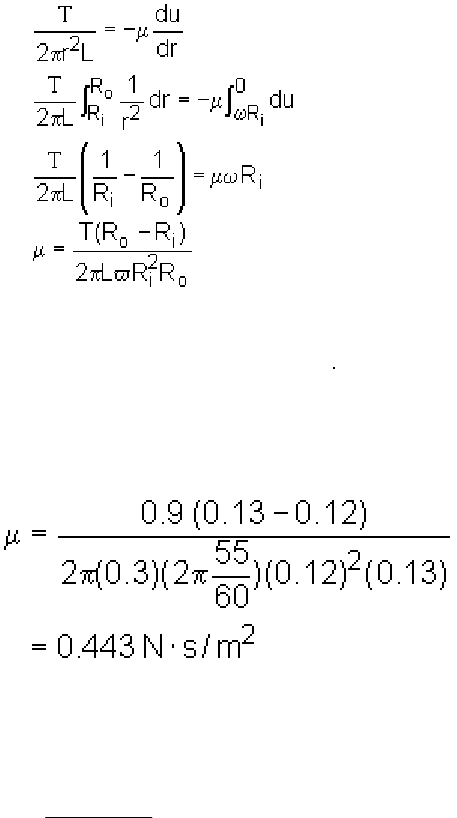Study Guides (400,000)
US (230,000)
LSU (10,000)
CE (200)
CE 2200 (30)
All (30)

# Exam 1 - Solution

Department
Civil Engineering
Course Code
CE 2200
Professor
All

This preview shows pages 1-3. to view the full 11 pages of the document.1
Solutions to CE2200 Exam #1
1. Choose the correct answer from the following options (20 pt: 4 points each):
(1) At boundaries, fluid particles adhere to the walls, and so their velocities are
zero relative to the wall. This characteristic is referred to as
(A) surface tension; (B) bulk modulus of elasticity; (C) no-slip condition.
(2) How many meters of water are equivalent to 760 mm Hg (in terms of
pressure)? SHg = 13.6.
(A) 13.60; (B) 10.34; (C) 0.76.
wh = Hg 0.76 h = 0.76(Hg/w) = 0.7613.6 (m)
(3) The center of pressure is always the centroid.
(A) below; (B) above; (C) coincident with.
(4) The dimension of force F is .
(A) ML/T2; (B) lbf; (C) N.
(5) Which of the following is the formula for the gage pressure within a very
small spherical droplet of water?
(A) p = /(2r); (B) p = 2/r; (C) p = 4/r.
2r = pr2 p = 2/r

Only pages 1-3 are available for preview. Some parts have been intentionally blurred.2
2. (20 pt): Students are given a simple device called a rotational cylindrical viscometer,
as shown in Fig. 1, and asked to determine the viscosity of an unknown liquid. The
outer cylinder is fixed while the inner cylinder is rotating at a constant angular speed
of by applying a torque T. (A) Derive an equation for the viscosity in terms of
angular velocity, , torque, T, submerged inner cylinder height, L, inner cylinder
radius, Ri, and outer cylinder radius, Ro; (B) Calculate the viscosity value when =
55 rev/min, T = 0.9 N m, L = 0.3 m, Ri = 0.12 m and Ro = 0.13 m.
SOLUTION:
Step-1: Find the dynamic viscosity of the fluid: = ?
Step-2: Schematic as shown in the figure.
Velocity Profile of the Fluid between the Gap of the Cylinders
Step-3: Assumptions: (1) The liquid is a Newtonian fluid; (2) The gap between the
cylinders is small and the velocity profile is linear, i.e., du/dr u/r=U/(Ro - Ri); (3)
Neglect the end effects of the cylinders; and (4) the force (F) is perpendicular to its
moment arm (r), hence the torque is given by T = Fr.
Step-4: Equations
The torque is given by multiplying the force with its moment arm. That
is,
T = Fr
The force can be expressed in terms of the shear stress and area as
follows:
F = (2rL) = /r
Rearrange terms to yield

Only pages 1-3 are available for preview. Some parts have been intentionally blurred.3
= / 2r2L
According to Newton's law of viscosity for Newtonian fluids,
=  du/dr
The negative sign is inserted to indicate that u decreases as r increases. Note that the
velocity profile is assumed to be linear, as shown in the figure. The end conditions are
known and are given by: u = Ri at r = Ri and u = 0 at r = Ro.
Equating the above two equations and integrating from
r = Ri to Ro:
Step-5: Known parameters
= 55 rev/min, T = 0.9 N m, L = 0.3 m, Ri = 0.12m and Ro = 0.13 m.
Step-6: Calculations
Substitution of the known values into the derived equation for dynamic viscosity yields
the viscosity of the liquid
Step-7: Discussion
Based on the assumptions, the dynamic viscosity can also be approximated as:
2
3s/mN 480.0
2
)(
i
io
RL
RRT
The thinner the gap layer is, the more accurate the solution is.